# HBSE 10th Class Science Important Questions Chapter 13 Magnetic Effects of Electric Current

Haryana State Board HBSE 10th Class Science Important Questions Chapter 13 Magnetic Effects of Electric Current Important Questions and Answers.

## Haryana Board 10th Class Science Important Questions Chapter 13 Magnetic Effects of Electric Current

Question 1.
Explain electromagnetism or electrodynamics (For in formation only).
1. From several experiments, it was proved that magnetic field can be produced through electricity and electric current can be produced through magnetic field. This effect of electricity on magnet is called ‘magnetic effects of electric current’ (The name of our chapter).

2. Thus, it was accepted that electricity and magnetism are associated with each other.

3. This branch of Physics that covers universal study of electricity and magnetism is called electromagnetism or electrodynamics.

4. The principles of electromagnetism are widely used in loud speaker, electric motor, magnetic train, computer hard disk, communication, etc.Question 2.
What is magnet and magnetism?
Substances that have property of attracting metals such as in, nickel, cobalt, etc. are called magnets. This property of attraction possessed by magnets is called magnetism.

Question 3.
What is a bar magnet? What do you mean by magnetic poles?
1. A magnet in the shape of a bar having two magnetic poles, namely the north pole and the south
pole is known as a bar magnet.
2. The parts of the magnet where the magnetic effect seems to be concentrated are called magnetic poles. Poles are usually located at the ends of the magnet. Hence, magnetism of magnets is maximum at the poles.Question 4.
What is the magnetic field of a magnet? Explain with the help of a diagram.
Magnetic field: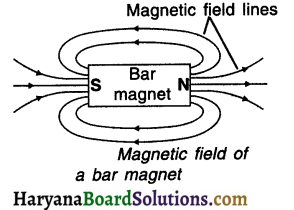• The region surrounding the magnet in which magnetic force can be experienced is called magnetic field.
• Field lines are drawn to describe the extent of the magnetic field.
• The magnetic field lines are the pictorial representation of a magnetic field.

Question 5.
Give the characteristics of magnetic field lines.
Characteristics of magnetic field lines:
(1) The magnetic field lines outside the magnet starts from the north pole (N) and reach the south pole
(2), whereas, inside the magnet, these lines start from south pole (S) and end at north pole (N).
(i) The inner and outer lines together form closed loops.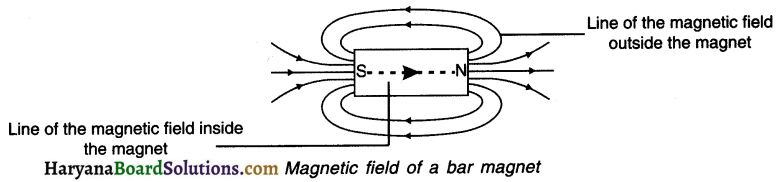(2) The region where the field lines are closer has a stronger magnetic field compared to the region where the field lines are farther.
(i) Thus the magnetic field is strong near the poles where the lines are quite close to each other.

(3) Magnetic field is a vector quantity. This means it has magnitude as well as direction.
(i) The tangent drawn at any point of a magnetic field line (the direction of magnetic needle at that point) shows the direction of magnetic field at that point.

(4) Magnetic field lines do not intersect each other.

• The imaginary pole at the top portion of the earth is called its Geographic North Pole.
• Similarly, the imaginary pole at the bottom portion of the earth is called its Geographic South Pole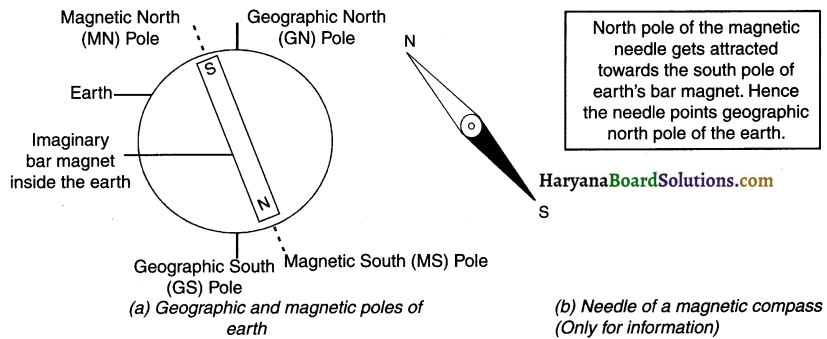Magnetic poles of the earth:

• Earth has magnetic field and so there are two magnetic poles of the earth.
• Near the Geographic North Pole of the earth lies its Magnetic North Pole.
• Whereas near the Geographic South Pole of the earth lies its Magnetic South Pole.

Earth as a magnet in its magnetic field:

• Although earth is in the shape of a sphere, imagine it as a very huge bar magnet.
• Naturally, this magnet will position itself in North-South direction. In other words, the South Pole o the earth’s bar magnet will get attracted to the Magnetic North Pole of the earth’s field.
• As a result, the north pole of our magnetic compass gets attracted towards the South Pole o Earth’s bar magnet and shows us north direction.Question 6.
What is a compass needle?
1. The needle-like structure that is found in the compass is called the compass needle. It is used to know the direction.
2. This needle is in fact a small bar magnet having two magnetic poles i.e. north pole and south pole. These two poles or ends approximately points north and south directions.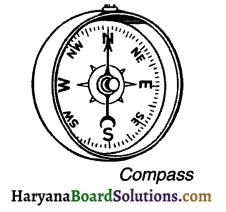Question 7.
No, the two magnetic lines of force cannot intersect.
Reason: If the two magnetic field-lines intersect then it would mean that at the point of intersection, the compass needle would point towards two directions which is not possible.

Question 8.
Why does a freely hanged magnet always become steady in North-South direction? OR Why does the needle of a magnetic compass always show north direction?
Earth behaves as a magnet.
1. Near the Geographic North Pole of the earth lies Magnetic North Pole of earth’s magnetic field, whereas near the Geographic South Pole of the earth lies Magnetic South Pole.
2. If you suspend a bar magnet freely or if you hold compass in your hand, the magnet will indicate the geographic north direction of the earth.

Reason:
Since unlike poles attract each other, the north pole of the magnet that you have gets attracted towards the Magnetic South Pole of the earth (i.e. Geographic North Pole) and south pole of the magnet gets attracted towards the Magnetic North Pole of the earth (i.e. Geographic South Pole).

Question 9.
Give inferences/conclusions of Oersted’s experiment.
(a) When electric current is passed through a conducting wire, the conductor starts behaving like a magnet and a magnetic field is produced around it.
(b) Magnetism and electric current are interrelated with each other.

Question 10.
How can it be shown that a magnetic field exists around a wire through which a direct electric current is passing?
1. When we bring the magnetic needle or say compass near the current carrying wire, the needle aligns itself perpendicular to the wire.
2. If we reverse the direction of the current the needle will also get reversed. These two acts confirm the presence of magnetic field around current carrying wire.Question 11.
State the factors on which magnetic field occurred due to a linear i.e. straight conductor (wire) carrying current depends on.
The magnetic field formed due to a linear (straight) conductor carrying current depends upon the following two factors:

• The magnetic field produced due to the current passing in the conductor is proportional to the electric current i.e. magnetic field intensity αelectric current.
• The magnetic field intensity goes on decreasing as the distance from the conductor increases i.e. magnetic field intensity α 1/distance.

Question 12.
Explain the ‘Right Hand Thumb Rule’ to know the direction of magnetic field.
Direction of magnetic field:
The direction of magnetic field associated with the electric current can be found with the help of the ‘Right Hand Thumb Rule’.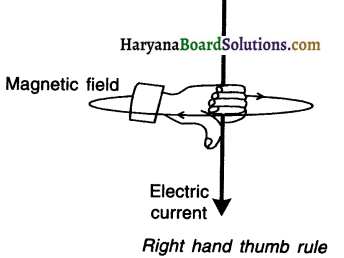1. For knowing the direction of magnetic field, imagine that you are holding the conducting wire in your right hand so that your thumb points in the direction of the current (I), then the direction in which your fingers encircle the wire will give the direction of magnetic field around the wire.
2. The wrapped fingers demonstrate closed loops.

Question 13.
State right hand thumb rule to find the direction of the magnetic field around a current carrying conductor. How will this field be affected on
(i) Increasing the current through the conductor?
(ii) Changing the direction of flow of current?
Direction of magnetic field:
The direction of magnetic field associated with the electric current can be found with the help of the ‘Right Hand Thumb Rule’.1. For knowing the direction of magnetic field, imagine that you are holding the conducting wire in your right hand so that your thumb points in the direction of the current (I), then the direction in which your fingers encircle the wire will give the direction of magnetic field around the wire.

2. The wrapped fingers demonstrate closed loops.

• Since magnetic field α I, the strength of magnetic field increases on increasing the current through the conductor.
• On changing the direction of flow of current, the direction of the magnetic lines of force is reversed.Question 14.
Discuss the magnetic field resulting from a current carrying circular ring (loop or coil). OR
Give an idea of magnetic field produced due to a current carrying loop of wire.
1. Bend a linear conductor (wire) in a circular shape to form a loop.
2. Connect this loop in the circuit and pass electric current through it.
3. By using the ‘Right Hand Thumb Rule’, we can find out the direction of magnetic field.
4. As shown in the figure, the magnetic field lines originating from the loop are represented by concentric circles.
5. These circles become larger and larger as we move away from the wire.
6. On reaching the centre of the loop, the arcs of these circles appear as straight lines and are closer to each other signifying a stronger magnetic field.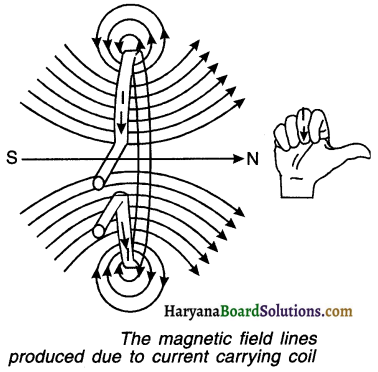Hence, we can conclude that the magnetic field B produced at the centre of the coil is —
1. Directly proportional to the current (I) flowing through it i.e. B α I
2. Inversely proportional to the radius (r) of the loop i.e. B α 1/r
3. Directly proportional to the total number of turns N of the coil i.e. B α N. This means if a ring is made up of closely spaced N turns, the magnetic field at the centre of the ring will be N times stronger.
Thus, magnetic field intensity $$\mathrm{B} \propto \frac{(\text { No. of turns } \mathrm{N})(\text { Electric current } \mathrm{I})}{(\text { Radius of ring } \mathrm{r})}$$

Question 15.
On which factors does the magnetic field produced at the centre of current carrying circular loop depends?
1. Bend a linear conductor (wire) in a circular shape to form a loop.
2. Connect this loop in the circuit and pass electric current through it.
3. By using the ‘Right Hand Thumb Rule’, we can find out the direction of magnetic field.
4. As shown in the figure, the magnetic field lines originating from the loop are represented by concentric circles.
5. These circles become larger and larger as we move away from the wire.
6. On reaching the centre of the loop, the arcs of these circles appear as straight lines and are closer to each other signifying a stronger magnetic field.

Hence, we can conclude that the magnetic field B produced at the centre of the coil is —
1. Directly proportional to the current (I) flowing through it i.e. B α I
2. Inversely proportional to the radius (r) of the loop i.e. B α 1/r
3. Directly proportional to the total number of turns N of the coil i.e. B α N. This means if a ring is made up of closely spaced N turns, the magnetic field at the centre of the ring will be N times stronger.
Thus, magnetic field intensity $$\mathrm{B} \propto \frac{(\text { No. of turns } \mathrm{N})(\text { Electric current } \mathrm{I})}{(\text { Radius of ring } \mathrm{r})}$$Question 16.
How can we increase the strength of the magnetIc field produced at the centre of current carrying circular loop?
We can we increase the strength of the magnetic field produced at the centre of current carrying circular loop by –

• Increasing the number of turns N of the coil or
• Increasing the current (I) through the coil or
• Decreasing the radius (r) of the coil.

Question 17.
What is a solenoid? Give the characteristics of magnetic field resulting from solenoid.
1. A long metal wire turned several times to form the structure of a coiled cylinder is known as a solenoid.
2. Figure (b) shows a solenoid connected to a circuit.
3. Let us assume that this solenoid has ‘N’ number of loops (i.e. N turns of coil).
4. On passing electric current, a magnetic field is produced inside the solenoid.
5. The magnetic field resulting due to N turns will be N times stronger than the magnetic field resulting by each circular coil.
6. This is because, in each turn, the direction of the current is same.
7. As a result, the magnetic field due to the current if each turn will be added.
8. This results in generation of a very strong magnetic field inside the solenoid.
9. On comparing figure (b) and figure (c), we can conclude that the magnetic field produced by a solenoid is quite similar to the magnetic field produced by a bar magnet.
10. Here, one end of the solenoid acts as a north pole while the other end acts as a South Pole.
11. The magnetic field produced due to a solenoid is dependent on the number of turns (n) and the current (I) flowing through it.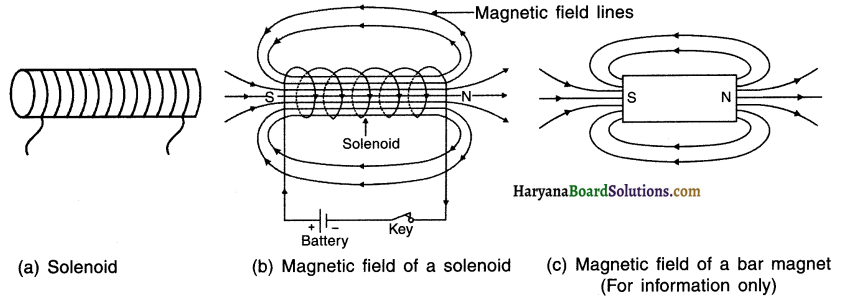12. On placing an rnn-like metal (e.g. large iron nails) inside the solenoid, its magnetic field becomes stronger.
13. On passing electric current through a solenoid, it behaves as a temporary magnet.
14. Such magnets are called ‘electromagnets’.

Question 18.
Draw a diagram to show magnetic field of a region which is uniform.
Uniform magnetic field in a given region can be shown through straight lines parallel to each other. The lines have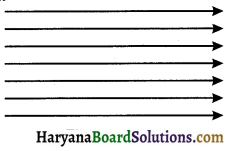Question 19.
State the factors on which the strength of the magnetic field produced by a current carrying solenoid depends.
The factors on which the strength of the magnetic field produced by a current carrying solenoid depends are —
(a) Number of turns (N) in the solenoid:
(i) More the number of turns (N) in the solenoid, stronger will be the magnetic field produced.

(b) Strength of the current (I) :
(i) Greater the current (I) passing through solenoid, stronger will be the magnetic field produced.

(c) Nature of core material:
(i) If material such as a soft iron cylinder is used in making the solenoid core, the magnetic field will be quite strong.Question 20.
What is an electromagnet? State the principle on which it works. Also state the factors on which strength of an electromagnet depends.
Electromagnet:
1. A soft iron core placed inside a solenoid behaves like a powerful magnet when a current is passed through solenoid. Such a magnet (with magnetic field around) is called an electromagnet.
2. The electromagnet works on the pnnciple of magnetic effect of electric current.

Factors on which the strength of the electromagnet depends:
(a) Direction of the current and
(b) Direction of the magnetic field.

• Magnitude of the force is highest when the direction of the current is perpendicular to the direction of magnetic field. At this arrangement the magnet will make maximum displacement.
• No force is exerted on the rod when the direction of electric current is the same as that of the magnetic field or is opposite to that of the magnetic field.

Question 21.
What do you mean by current-carrying conductor placed In an external magnetic field?
When a conductor is connected in an electric circuit it is called ‘current carrying conductor’. When we bring a strong magnet near this conductor such that the conductor falls within the magnetic field of the magnet, we say current-carrying conductor lies within a magnetic field.

Question 22.
Why does a current-carrying conductor experience a force in a magnetic field?
1. When electric current flows through a conductor, the conductor produces a magnetic field around it.
2. If we bring a magnet near this conductor, the conductor’s magnetic field will exert a force on the magnet. At the same time, the magnet also exerts an equal and opposite force on the current carrying conductor.
3. It was French scientist Andre Ampere who experimentally demonstrated the presence of force exerted by a magnet.

Question 23.
State the factors on which the forces acting on a current-carrying conductor placed in a magnetic field depend.
The forces acting on a current-carrying conductor placed in a magnetic field depends on:
(a) Direction of the current and
(b) Direction of the magnetic field.

• Magnitude of the force is highest when the direction of the current is perpendicular to the direction of magnetic field. At this arrangement the magnet will make maximum displacement.
• No force is exerted on the rod when the direction of electric current is the same as that of the magnetic field or is opposite to that of the magnetic field.

Question 24.
State and explain Fleming’s left hand rule. OR Name and state the rule to determine the direction of a force experienced by a straight conductor carrying current placed In a magnetic field which is perpendicular to it.
1. Arrange your left hand such that the fore finger, the center finger and thumb remain at right angle to each other.
2. Adjust your hand in such a way that the forefinger points in the direction of magnetic field and the centre finger points in the direction of current, then the direction in which the thumb points will be the direction of magnetic force.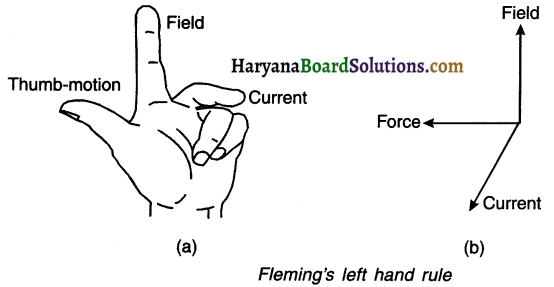3. Arrange your left hand such that the fore finger, the center finger and thumb remain at right angle to each other.
4. Adjust your hand in such a way that the forefinger points in the direction of magnetic field and the centre finger points in the direction of current, then the direction in which the thumb points will be the direction of magnetic force.Question 25.
An electron enters a magnetic field at right angles to it, as shown in figure. The direction of force acting on the electron will be: (Textbook Example 13.2)
(a) to the right
(b) to the left
(c) out of the page
(d) into the page
Reason:
1. As can be seen in the figure, the magnetic field is uniform and directed from left to right horizontally in the plane of paper.
2. The electron moves perpendicular to the magnetic field in vertically downward direction and in the plane of paper.

Question 26.
What is an electric motor? State some of its uses.
An electric motor is a rotating device which converts electric energy into mechanical energy.
Uses: It is used in electric fan, mixer-grinder, washing machine, DVD players, etc.

Question 27.
Describe the principle, construction and working of an electric (DC) motor along with a figure.
Electric motor : An electric motor is a device which converts electric energy into mechanical energy.
Principle :
1. When a current carrying conductor (or a coil) is placed in a magnetic field, the conductor experiences force and it rotates.
2. Electric motor works on the same principle.

Construction :
1. An electric motor, as shown in figure, consists of a rectangular coil ABCD made from insulated copper wire.
2. The coil is placed between the two poles of a magnetic field such that the arm AB and CD are perpendicular to the direction of the magnetic field.
3. The ends of the coil are connected to the two halves P and Q of a split ring.
4. The inner sides of these halves are insulated and attached to an axle such that they can rotate easily.
5. The external conducting edges of P and Q touch two conducting stationary brushes (i:e. carbon strips) X and Y, respectively. Construction of an electric motor
6. Finally, the terminals of the battery are connected with brushes ‘X’ and ‘Y’.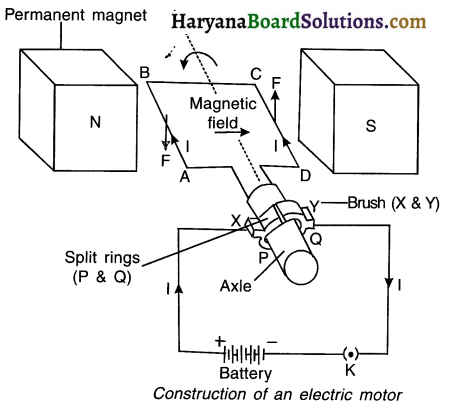Working: Current in the coil ABCD enters from the source battery through conducting brush X and flows back to the battery through brush Y.

1. The current in arm AB of the coil flows from A to B, whereas in arm CD it flows from C to D, i.e. opposite to the direction of current through arm AB.

2. On applying Fleming’s left hand rule, we find that the force acting on arm AB pushes it downwards while the force acting on arm CD pushes it upwards.

3. As a result, the coil and the axle O, mounted free to turn about an axis, rotate anti-clockwise.

4. At half rotation, Q makes contact with the brush X and P with brush Y. So the current in the coil gets reversed and flows along the path DCBA.

5. The reversal of current also reverses the direction of force acting on the two arms AB and CD. Thus the arm AB of the coil that was earlier pushed down is now pushed up and the arm CD previously pushed up is now pushed down.

6. Hence, the coil and the axle rotate half a turn more in the same direction. The reversing of the current is repeated at each half rotation, giving rise to a continuous rotation of the coil and to the axle.
Uses: The electric motor is used in appliances such as electric fan, mixer, washing machine, CD player, etc.Question 28.
What are the special features of a commercial electric motor?
(1) A commercial motor uses an electromagnet in place of a permanent magnet.
(2) It has large number of turns of the conducting wire in the current-carrying coil.
(3) It has a soft iron core on which the coil is wound. The soft iron core along with the coil is called armature. This enhances the power of the motor.

Question 29.
1. What is electromagnetic induction and induced current? Who gave this concept?
2. On what factors does the value of induced current produced in a circuit depend?
3. Name the rule used to determine direction of induced current.
4. State one practical application of this phenomenon in everyday life.
1. When the magnetic line of force passing through a closed circuit change, a voltage and hence a current is induced in it. This phenomenon is called electromagnetic induction.

• The voltage produced is called induced force (e.m.f.) and there current is called induced current.
• English physicist Michael Faraday gave this concept in 1831.

2. The value of current induced in the circuit depends on the rate of change of magnetic flux linked with the closed circuit.
3. Fleming’s right hand rule is used to find the direction of induced current.
4. Electromagnetic induction is used in electric generators.

Question 30.
With the help of an activity, explain the method of inducing electric current in a coil with a moving magnet.
Aim: To study the phenomenon of electromagnetic induction.

Procedure:
1. Take a coil of wire AB having a large number of turns.
2. Connect the ends of the coil to a galvanometer as shown in Figure.
3. Take a strong bar magnet and move its north pole towards the end B of the coil. Do you find any change in the galvanorneter needle?
5. There is a momentary deflection in the needle of the galvanometer, say to the right. This indicates the presence of a current in the coil AB. The deflection becomes zero the moment the motion of the magnet stops.
6. Now withdraw the north pole of the magnet away from the coil. Now the galvanometer is deflected toward the left, showing that the current is now set up in the direction opposite to the first.
7. Place the magnet stationary at a point near to the coil, keeping its north pole towards the end B of the coil. We see that the galvanometer needle deflects toward the right when the coil is moved towards the north pole of the magnet. Similarly the needle moves toward left when the coil is moved away.
8. When the coil is kept stationary with respect to the magnet, the deflection of the galvanometer drops to zero. What do you conclude from this activity?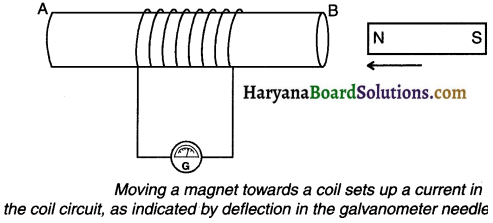Observation and conclusion:

• When we move the magnet towards away from the coil or when we move the coil towards/away from the magnet, the magnetic lines of force passing through the closed coil gets charged.
•  This induces potential difference in the coil which in turn induces current in the circuit.
• We can observe the presence of current in the galvanometer needle.
• The needle shows no movement when both, the coil and the magnet remain stationary.
• We conclude that when there is no change in the magnetic lines of force passing through the coil, no potential difference is induced and hence no current is induced.

(For information only:
Note: Although, as mentioned above, students need to write activity 13.8 for this answer, the description given below will help them to understand the concept of electromagnetic induction in a better way.

• In 1831, a British scientist Michael Faraday gave the principle of electromagnetic induction.
• He, through his experiments, showed that a magnetic field can produce electric current.
• As shown in the figure, a loop of conducting wire is connected to a galvanometer.
• Galvanometer indicates the status of current.Situation 1 :
1. As shown in figure (a), we keep the bar magnet in a stationary position.
2. Here, the pointer of galvanometer remains stationary which indicates that there is no flow of current in the loop.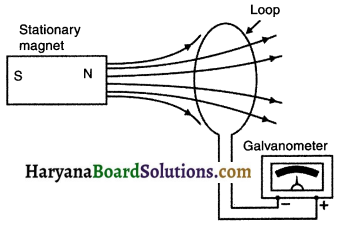Situation 2 :
1. When we quickly move the magnet towards the loop, the pointer of the galvanometer shows deflection. [Figure (b)]
2. This indicates that current is induced in the coil due to the motion of the magnet.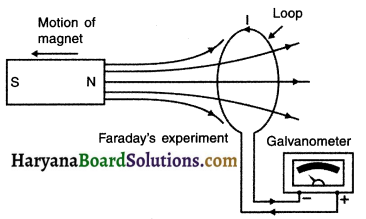Situation : 3 :
1. Again, when we move the magnet away in the opposite side from the loop, the pointer of the galvanometer gets deflected in the opposite direction. [Figure (c)]
2. This indicates that current is induced in the opposite direction.Conclusion :

• We can conclude from the above experiment that current is induced in the loop when there is a change in the position of the bar magnet.
• Here, the current is said to be induced by the motion of the magnet.
• Hence, it is termed as ‘induced electric current’ or electromagnetic induction.)

Question 31.
By which law can the direction of induced electric current be obtained? Explain the law.
1. The direction of electric current can be found out with the help of Fleming’s right hand rule.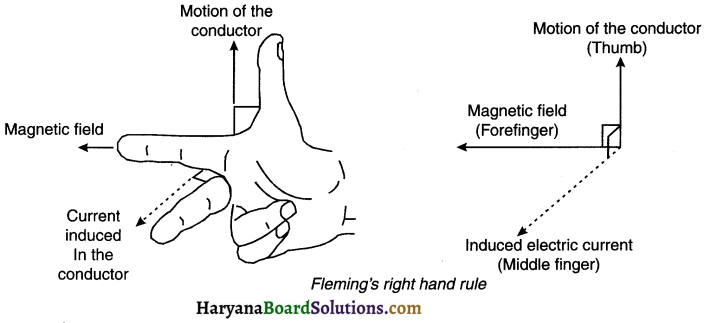2. Arrange the forefinger, middle finger and thumb of a right hand at right angle to one another.
3. Adjust the forefinger in the direction of magnetic field, and thumb pointing in the direction of motion of conductor.
4. The direction of middle finger will then indicate the direction of induced electric current.Question 32.
What is galvanometer? Explain.
Galvanometer: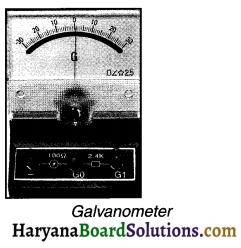1. A galvanometer is an instrument that can detect the presence of current in a circuit i.e. whether the current is flowing in the circuit or not.
2. If no current is flowing in the circuit, the pointer of galvanometer shows 0 (zero).
3. If the current is flowing, the pointer may deflect left or right depending upon the current.

Question 33.
What is an electric generator? State its principle.
1. A device that converts mechanical energy into electrical energy is called an electric generator.
2. The electric generator works on the principle of electromagnetic induction.

Question 34.
Describe the principle, construction and working of an electric generator along with a figure.
Electric generator:
An electric generator is a device which converts mechanical energy into electric energy.

Principle:
The electric generator works on the principle of electromagnetic induction.

Construction:
(Note: The construction and working shown here is of an Alternating Current (AC) generator.)
1. An AC electric generator, as shown in the figure consists of a rotating rectangular coil ABCD placed between the two poles of a permanent magnet.
2. The two ends of this coil are connected to the two rings R1and R2. The inner side of these rings are insulated.
3. The two conducting stationary brushes B1 and B2 are kept pressed separately on the rings R1 and R2 respectively.
4. The two rings R1 and R2 are internally attached to an axle.
The axle may be mechanically rotated from outside to rotate the coil inside the magnetic field.
Outer ends of the two brushes are connected to the galvanometer to show the flow of current in the given external circuit.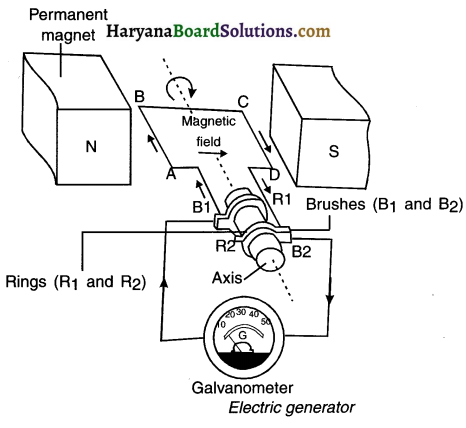Working:
1. Suppose the axle attached to the two rings is rotated such that the arm AB moves up (and the arm CD moves down) in the magnetic field produced by the permanent magnet. Then the coil ABCD gets rotated clockwise.

2. By applying Fleming’s right-hand rule, we can determine that the induced currents are set up in these arms along the directions AB and CD. Thus an induced current flows in the direction ABCD or say from B2 to B1.

(Note: If there are larger numbers of turns in the coil, the current generated in each turn adds up to give a large current through the coil.)

3. After half a rotation, arm CD starts moving up and AB, down. As a result, the directions of the induced currents in both the arms change. This gives rise to the net induced current in the direction DCBA. The current in the external circuit now flows from B1 to B2.

4. Thus after every half rotation, the polarity of the current in the respective arms changes. Such a current, which changes direction after equal intervals of time, is called an alternating current (abbreviated as AC).Question 35.
What changes do we need to make in the set-up of a generator if the generator is to produce Direct Current (DC)?
1. In order to get a direct current (DC), a split-ring type commutator must be used instead of a full-ring as seen in AC generators.
2. By doing so, one brush all the time remains in contact with the arm moving up in the field, while the other remains in contact with the arm moving down.
3. This way a unidirectional current is produced. Such a generator is called a DC generator.

Question 36.
State the uses of electric generator.
1.  Electricity is generated by allowing the turbines of electric generators to move through the force of water in the dams.
2. In wind mills, the electricity is produced by rotating its coil through wind energy.
3. A diesel engine is also used to rotate the coil of the generator placed in hospitals, circus, fun-fairs, etc.

Question 37.
Explain DC and AC current.
There are two types of electric current, namely DC current,and AC current.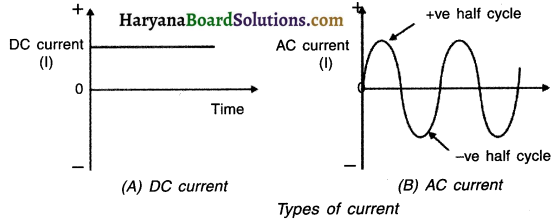(A) DC current :

• The current that always flows in the same direction is known as direct current (DC).
• In a battery, the current flows in one fix direction i.e. from positive (+) to negative (-) terminal.
• Hence, current obtained from sources like battery or DC generators is known as DC current.
• In DC current, the voltage and current always remain constant.
• Batteries used in radio/cell phone, watch, laptop, etc. works on DC.

(B) AC current :
1. The current that changes direction after equal intervals of time is known as alternating current (AC).
2. Such a current is obtained from AC generators.
3. In an AC generator, the voltage and current change their directions between positive (+) and negative (-) terminals periodically.
4. In our country, AC voltage of 220V and 50 Hz is used for domestic purpose.
5. The AC voltage current changes its direction 100 times in one second and hence its frequency is $$\frac{100}{2}$$ = 50 Hz.
6. Appliances such as fan, tubelight, TV, refrigerator, etc. run on AC Current.
7. Producing and transposing alternate current (AC) er a longer distance is quite cheap as compared to DC.

Question 38.
Why is alternating current preferred over direct current? List any three reasons.
Advantages of alternating current (AC) over direct current (DC):

• AC current is cheaper than DC current.
• Fluctuations in voltage can be easily controlled with the help of transformer.
• AC current can be transmitted to distant places without much loss in the power.Question 39.
Draw a schematic diagram of household domestic wiring and explain its main features.
1. The current that comes to our house from the power stations is Alternate Current (AC).
2. From power station, this power is transmitted to our houses through thick underground cables or overhead electric poles and cables.
3. These wires enter our house through main electrical boards of our house. It is also called main supply or simply mains.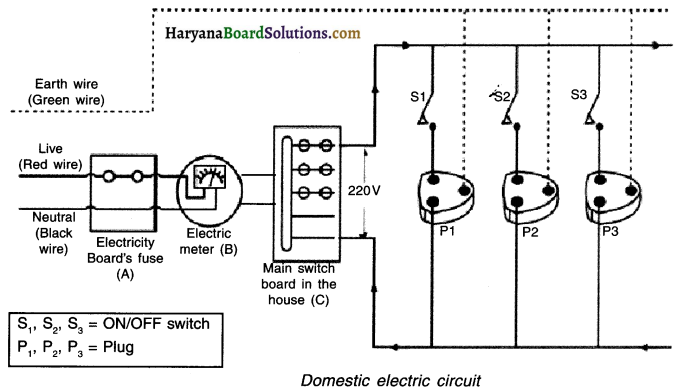4. Three main wires enter the main board of our house. They are:

• Wire with red coloured insulation. This wire is known as live wire or positive.
• Wire with black coloured insulation. This wire is known as neutral wire or ‘negative’.
• Wire with green coloured insulation. This wire is known as earth (or earthing) wire.

5. A voltage of 220V with 50 Hz frequency is supplied to our houses between live and neutral wires. All the above wires first enter the main fuse in the electric board or say Electricity Board’s fuse (A), then to electrical meter (B) and then to the main switch board (C) of our house.

6. In the main switchboard of the house, different types of fuses are installed as per the need of the appliances to be used. The earth-wire or ‘earthing’ provides a low-resistance conduction path for the current.

7. The main wires are distributed among these fuses and from there the electrical power is distributed in the house accordingly.

8. Moreover, two separate circuits are used for line with 5A and 15A current respectively to avoid over loading.

9. The 5A line is used for running appliances with less power ratings such as fan, T.V., tube lights, etc. The 15A line is used for running appliances with higher power rating such as air conditioner, geyser, etc.

10. Each appliance has a separate switch to ‘ON’/‘OFF’ the flow of current through it.

11. The entire wiring of house is done in a parallel connection so that each appliance gets equal potential difference.Question 40.
State how a wire is earthed and discuss the need of earthing.
1. The earthing wire is a green colour wire connected to a metal plate. The metal plate is buried deep in the earth near our house.

2. In case, if the live wire touches the metal case of the appliance (i.e. in case of leakage of current), then due to earthing, the current will directly pass into the earth without causing electrical shock to the user.

3. To avoid electrical shocks, metal appliances such as toaster, electric iron, refrigerator, table fan, etc. are connected to the earth wire. Thus, a person is saved from any electrical shocks occurring due to leakage of current.

Question 41.
Name the three wires that are used in our household circuits along with their colours.
1. Live (positive) wire with red coloured insulation.
2. Neutral (negative) wire with black coloured insulation.
3. Earth wire (earthling) with green coloured insulation

Question 42.
What is short circuit? Explain.
1. If positive (live) and negative wires touch each other accidently-such a situation is called short-circuit.
2. Short circuit may occur if the insulating layers of wires or appliances tear or are defective.
3. Under these circumstances, the total resistance of circuit suddenly decreases and an excessive electric current flows as per Ohm’s law.
4. This produces a lot of heat and a spark at the point of short circuit.
5. Short circuit may also lead to fire.

Question 43.
What is a fuse? Mention the usefulness of fuse in a domestic electrical circuit.
Fuse : A fuse is a safety device made out of small metallic strip with a low melting point. It works on the heating effect of the electric current.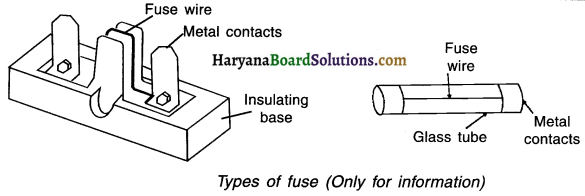Construction :

• A fuse consists of a base made out of porcelain (as shown in first figure).
• There are two metal terminals on this base.
• A conducting wire with low melting point is joined between these two terminals.

Working of fuse:

• When the electric current increases beyond the rating (capacity) of the fuse, the fuse wire melts, the fuse burns and breaks the circuit from that point and prevents damage of the appliances.
• Fuse wire of appropriate thickness improves its efficiency to prevent damage.Question 44.
Short-circuit:
1. At times due to over-heating or damaged wires, the plastic insulation of live wire and negative wire get touched. When these two wires come in contact that is when they touch each other it leads to a phenomena called short-circuit.

2. The resistance of the circuit becomes almost zero and hence a very large amount of current starts flowing in the circuit. This may cause a spark and even fire.

• The current flowing in domestic wiring depends upon the power ratings of the appliances being used.
• If a large number of electrical appliances are switched on simultaneously, they start drawing large amount of current from the mains. When the current drawn exceeds the safety limit it is called over loading.

Question 45.
What are magnetic field lines? How is the direction of a magnetic field at a point determined?
1. The region surrounding the magnet in which magnetic force can be experienced is called magnetic field. Field lines are drawn to describe the extent of the magnetic field. The magnetic field lines are the pictorial representation of a magnetic field.

2. The direction of magnetic field is determined by placing a small compass needle. The direction of deflection of N-pole of the compass needle gives the direction of the magnetic field at that point.

Question 46.
What is a magnetic compass? Explain with the help of a diagram.
Magnetic compass: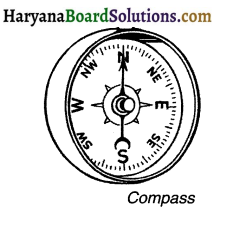• Magnetic compass is a small instrument which helps us to locate the direction.
• The compass has a dial on which directions namely North N, South S, East E and West W are marked.
• There is a magnetic needle on the axis of this dial which can rotate freely.
• The needle always points towards the North-South direction.
• Thus, if one holds the compass in his hand, the needle will show the north and the south directions. Based on this, one can determine other directions also.Question 47.
(a) What is the direction of magnetic field lines inside a bar magnet and outside of it?
(b) What does the degree of closeness of the field lines represent?
(a) The direction of the magnetic field lines is from N-pole to S-pole outside of the magnet and from S-pole to N-pole inside the magnet.
(b) The degree of closeness of the field lines gives a measure of the strength of the magnetic field.

Question 48.
A student performs an experiment to study the magnetic effect of current around a current carrying straight conductor. He reports that —
(i) The direction of deflection of the north pole of a compass needle kept at a given point near the conductor remains unaffected even when the terminals of the battery sending current in the wire are interchanged.
(ii) For a given battery, the degree of deflection of N-pole decreases when the compass is kept at a point farther away from the conductor. Which of the above observations of the student is incorrect and why?
Observation (i) is incorrect.
Reason: When the direction of flow of current is changed, the direction of the magnetic field and hence the direction of force on the compass needle also gets changed.

Question 49.
What change in the deflection of the compass needle placed at a point near current carrying straight conductor shall be observed if the –
(a) Current through the conductor is increased?
(b) Direction of current in the conductor is reversed?
(c) Compass is moved away from the conductor?
(a) The deflection of the compass needle increases because in the strength of the magnetic field increases.
(b) The direction of deflection of the compass needle gets reversed because the direction of magnetic field is reversed.
(c) The deflection of the compass needle decreases because the strength of the magnetic field has decreased.

Question 50.
A current carrying straight conductor is placed in east-west direction. What will be the direction of the force experienced by this conductor due to earth’s magnetic field? How will this force get affected on:
(i) Reversing the direction of flow of current?
(ii) Doubling the magnitude current?
The direction of earth’s magnetic field is from geographical south to geographical north. According to Fleming’s left hand rule, the current carrying straight conductor placed in east-west direction will be deflected downwards.

• So, if we reverse the direction of flow of current, the conductor will be deflected in the upward direction.
• If the magnitude of current is doubled, the magnitude of the force will also be doubled.

Question 51.
A metallic conductor is suspended perpendicular to the magnetic field of a horse shoe magnet. The conductor gets displaced towards left when a current is passed through it. What will happen to the displacement of the conductor if the:
1. Current through it is increased?
2. Horse shoe magnet is replaced by another stronger horse shoe magnet?
3. Direction of current through it is reversed?
1. Displacement of the conductor towards left will increase.
2. Displacement of the conductor towards left will increase.
3. The conductor is displaced towards right.

Question 52.
What happens to the force acting on a current carrying conductor placed in a magnetic field when –
(a) Current in conductor increases.
(b) Length of the conductor increases.
(c) Direction of the current and magnetic field both are reversed.
(d) Direction of the current is reversed without changing the direction of magnetic field.
(a) Force F is directly proportional to current I i.e. F α = I. So, force on the conductor will increase.
(b) Force on the conductor increases, as F α L.
(c) Force on the conductor remains same both in magnitude and direction.
(d) The direction of force on the conductor is reversed.Question 53.
State Oersted’s and Ampere’s discoveries over current-carrying conductor.
1. Oersted discovered that a current-carrying conductor exerts force on the magnet placed near the conductor.
2. Andre Ampere suggested that the reverse is also true i.e. the magnet also exerts equal and opposite force on the current-carrying conductor.

Question 54.
A coil made of insulated copper wire is connected to a galvanometer.
(i) What will happen to the deflection of the galvanometer if this coil is moved towards a stationary bar magnet and
(ii) Then moved away from it? Give reason for your answer and name the phenomenon involved.
(i) When the coil is moved towards the bar magnet, the galvanometer show deflection in one direction.
Reason: When the coil is moved towards the stationary bar magnet, the magnetic flux linked with it increases and current is induced in it. This can be verified with the deflection shown by the galvanometer.

(ii) When the coil is moved away from the coil, the galvanometer shows deflection in opposite direction.
Reason: When the coil is moved away from the magnet, the magnetic flux linked with it decreases and a current is induced in the coil in the opposite direction. The phenomenon involved is called electromagnetic induction.

Question 55.
What precautions should we take while making electrical connections? ((13.7))
Following precautions should be taken while using making electrical connections:

• We should see that the positive and the negative terminals of the electrical circuit do not come in contact directly.
• Care should be taken that the insulation of the wire is not torn.
• Proper earthing should be done as and where required.
• The wiring should be done as per standards.
• Separate lines i.e. 5A and 15A should be used as per the appliances.
• All the appliances of the house should not run on one fuse, rather they must be divided among various fuses in order to divide power load.

Question 56.

• Live wire and neutral come in direct contact.
• Sudden rise in supply voltage.
• Running too many heavy appliances like AC, oven, etc. for quite a long time.
• Connecting several appliances to a single socket.

Question 57.
An electric fuse of rating 3 A is connected in a circuit in which an electric iron of power 1 kW is connected which operates at 220 V. What would happen? Explain.
$$\mathrm{I}=\frac{\mathrm{P}}{\mathrm{V}}=\frac{1 \mathrm{~kW}}{220 \mathrm{~V}}=\frac{1000 \mathrm{~W}}{220 \mathrm{~V}}=4.55 \mathrm{~A}$$
The electric current required by the electric iron is more than the current that can flow through the fuse without it getting melt.
As a result, the circuit will get overloaded. This will melt the fuse wire and so electric current will not flow in the circuit.

Question 58.
In a house hold electric different appliances are connected in parallel to one another. Give two reasons.
An electrician puts a fuse of rating 15 A in that part of domestic electrical circuit in which an electrical heater of rating 1.5 kW, 220 V is operating. What is likely to happen in this case and why? What change, if any, needs to be made?

In a household electric circuit, different appliances are connected in parallel to one another due to the following reasons:

• In a parallel connection, the appliances can be operated independently i.e. can be switched on and off independently.
• Each appliance gets the same constant voltage.

The current that the electrical heater will draw can be calculated as follows:
$$I=\frac{P}{V}=\frac{1.5 \mathrm{~kW}}{220 \mathrm{~V}}=\frac{1500 \mathrm{~W}}{220 \mathrm{~V}}=6.8 \mathrm{~A}$$
The fuse is of 15 A whereas the current that the heater will draw is just 6.8 A. So, the fuse will not blow in case if sometimes a higher current flows in the circuit accidentally. So, no change is needed.Question 59.
Differentiate between AC and DC.

 DC current AC current DC constantly flows in same direction. AC changes direction after equal intervals of time. The value of this current remains constant with time. The value of this current changes with time. Its production is costly. Its production is cheaper. There is much loss of energy while transmitting DC over a large area. There is lesser loss of energy while transmitting AC over a large area.

Question 60.
Differentiate between electric motor and electric generator.

 Electric motor Electric generator It converts electrical energy into mechanical energy. It converts mechanical energy into electrical energy. Electric motor work son the principle that a current carrying loop kept in a magnetic field experiences force and hence gives mechanical energy. Electric generator works on the principle of electromagnetic induction. It is used in running fan, washing machine, mixers, etc. It is used in diesel generators, wind mills, etc.

Question 61.
Differentiate between Fleming’s left hand thumb rule and right hand thumb rule.

 Fleming’s left hand thumb rule Fleming’s right hand thumb rule 1. This rule is used to determine magnetic force on the conductor. This rule is used to determine the direction of introduced current. 2. Direction of thumb gives the direction of magnetic force. Direction of thumb gives the direction o motion of the conductor. 3. Direction of centre finger represents direction of electric current. Direction of centre finger represents induced current in the conductor.

Question 62.
Differentiate between permanent magnet and electromagnet.

 Permanent magnet Electromagnet It retains magnetism for very long time. It is a temporary magnet. So, it shows magnetic property as long as the current is passing through its coil. It has a weaker magnetic field. It can produce a very strong magnetic field. Its strength is fixed. Its strength can be changed. Polarity of this magnet cannot be changed. Polarity of electromagnet can be reversed.

Question 63.
Two brothers Sumit and Girish after studying about the working of water-pump in the school decided to understand the working of electric motor practically back home. They collected different parts used in an electric motor such as magnets, carbon brushes, armature, battery, switch, connecting wire, split-rings, etc. and assembled them to form an electric motor.

Questions:

1. State the principle how an electric motor works.
2. Is an electric motor needed for water-pump to work?
3. What values do you learn from this activity?

1. When a current-carrying coil is placed in a magnetic field, it experiences a force. The direction of this force is found by using the Fleming’s left-hand rule.
2. Yes. An electric motor is needed for a water pump to work.
3. The activity shows aptitude for practical demonstration and enthusiasm to apply class room learning practically.Question 64.
One day Dinesh wanted to iron his shirt for the office. When he took out the iron he saw that the plug was broken. The only plug that he had at home was a 2 pin plug. Dinesh fixed the black and red wire in the two pin plug and ironed his shirt.
After few days, his sister got a severe electric shock while ironing the clothes.

Dinesh immediately took her to the hospital for a check-up. Thankfully she was fine. Dinesh then called the electrician to find out what went wrong. He told that this situation could have been avoided if she would have connected the green wire also by using a three-pin plug Bharat learnt a lesson.

Questions:

1. What do you think the electrician would have told Dinesh?
2. Which wire did Dinesh disconnect while ironing the shirt? What is the use of this third wire?
3. What care should Dinesh take to avoid such mistakes?

1. The electrician would have told Dinesh that he should not use a two-pin plug if the appliance uses a three-pin plug. The current leaked from the iron and so Dinesh’s sister got an shock.
2. Dinesh disconnected the green coloured earth-wire. Although one can still run the appliance but, the earth wire protects one during leaked current and hence electric shock.
3. Dinesh should never run an appliance which runs on three-wire on a two-pin plug.

Very Short Answer Type Question :

Question 1.
What was the observation that proved a correlation between electricity and magnetism? Who was the first to observe this characteristic?
Hans Christian Oersted was first to observe that compass needle (a magnet) got deflected when an electric current passed through a conductor placed in vicinity. This observation proved a correlation between magnetism and electricity.

Question 2.
What does the direction of thumb indicate in the right hand thumbs rule? In what way this rule is different from Fleming’s left hand rule.
Thumb indicates the direction of electric current flowing through the conductor whereas the direction in Fleming’s left hand rule indicates the direction of force experienced by current carrying conductor in a magnetic field.

Question 3.
Mention any two characteristics of magnetic field lines.
(i) Magnetic field lines form a closed loop.
(ii) No two field-lines are found to intersect or cross each other.Question 4.
A magnetic compass needle is placed in the plane of paper near point A as show in figure. In which plane should a straight current carrying conductor be placed so that it passes through A and there is no change in the deflection of the compass? Under what condition is the deflection maximum and why?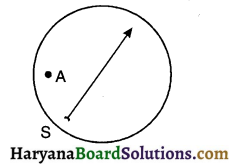The conductor should be placed horizontally on the plane such that it passes through point A. This way the magnetic field generated will be perpendicular to the plane. The compass placed near that point has the freedom to deflect in the horizontal direction only. Since the magnetic field produced is perpendicular, the compass needle will not experience any deflection.

Question 5.
Rahul is provided with an image of magnetic field and is asked to point out one of the regions with high magnetic strength. How can he identify the region with high magnetic strength just by looking at the image?
The region in the magnetic field where the field lines are concentrated i.e. are more has a high magnetic strength as compared to the region with scarce field lines.

Question 6.
No two magnetic field lines cross each other. Justify the statement.
If two magnetic field lines cross each other it means that the north pole of magnet placed at the point of intersection would exert force in two different directions which is never possible. Hence it is rightly said that no two magnetic field lines cross each other.

Question 7.
Given here is the image of a bar magnet and a magnetic compass placed in the field of the magnet. From the direction of the needle of compass identify the pole that is closer to Point ‘B’.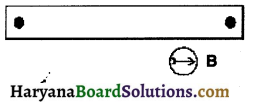South pole of the magnet is closer to point B.

Question 8.
On which physical quantities is the magnitude of magnetic field dependent? How?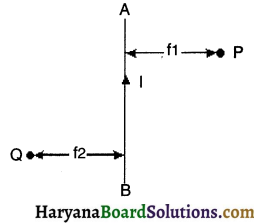Magnetic field of a current carrying conductor is directly proportional to the electric current flowing through it and inversely proportional to the distance from the conductor.

Question 9.
AB is a current conductor in the plane of the paper as shown in figure. What are the directions of magnetic fields produced by it at points P and Q? Given r1 > r2, where will the strength of the magnetic field be larger?
Using right hand thumb rule, the direction of magnetic field will be coming out of point Q and entering point P such that if viewed from point A of conductor the direction will be anti-clockwise. Since r1 > r2, and magnetic field strength is inversely proportional to distance, magnetic field will be stronger at point Q.Question 10.
It is established that an electric current through a metallic conductor produces a magnetic field around it. Is there a similar magnetic field produced around a thin beam of moving (I) alpha particles, (II) neutrons? Justify your answers.
Any charged particle in motion would generate a similar magnetic field because a charged particle in motion is nothing but an electric current.

• Alpha particle is a positively charged particle and hence it will generate a similar magnetic field.
• Neutron does not have any charge and hence it will not generate a magnetic field around it.

Question 11.
Given here is the image of magnetic field formed around the current carrying conductor. What would be the direction of current flowing through it?
Direction of current flowing through conductor is perpendicular to this horizontal plane and moving in upward direction.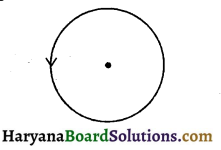Question 12.
Electric current Is flowing through a conductor In downward direction. What would be the direction of magnetic field produced around
the conductor? Which rule can be used to find out the direction of magnetic field?
The magnetic field produced will have a clockwise direction if viewed from top. Right hand thumb rule is used to identify the direction of magnetic field.

Question 13.
If there is a circular coil having ‘n’ turns, the field produced is ‘n’ times as large as the field produced by a single turn. Justify the statement.
As the current in each circular turn has the same direction, and the field produced due to each turn adds up to its magnitude, we can rightly say that magnitude of field produced in such coil is ‘n’ times as large as that produced by a single turn.

Question 14.
Draw a diagram of magnetic field around a solenoid. State one of the uses of solenoid.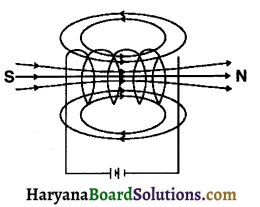Solenoid is used to magnetize a material like soft iron and convert it into an electromagnet.Question 15.
What observation did Andre Marie Ampere make on a magnet when it was placed in the vicinity of a current carrying conductor?
He observed that when a magnet is placed in the vicinity of a current carrying conductor, it exerts equal and…bpposite force on the conductor as the conductor exerts on the magnet.

Question 16.
On which physical quantities Is the direction of force on conductor kept in a magnetic field is dependent?
Direction of force on conductor depends upon the direction of the current flowing through the conductor and the direction of magnetic field.

Question 17.
What is Fleming’s left hand rule used for?
Fleming’s left hand rule is used to find out the direction of force on a current carrying conductor kept in a magnetic field.

Question 18.
A positively charged particle (alpha particle) projected towards west is deflected towards north by a magnetic field. State the direction of the magnetic field. Which rule did you use to find the direction?
The direction of the magnetic field is vertically upwards. The ruled used is Fleming’s left hand rule.Question 19.
State the principle on which electric motor works.
Electric motor works on the principle which says “when a current carrying conductor is placed in a magnetic field, the field exerts a force on the conductor which is perpendicular to the direction of the flowing current and the magnetic field.”

Question 20.
Provided here is the image of a conductor kept in a magnetic field. In which direction will conductor move if the current is applied from A to B ?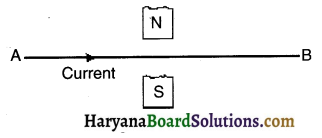The conductor will move in downward direction.

Question 21.
What is the function of carbon brushes in an electric motor?
The function of carbon brushes is to make the sliding contact with the split rings in order to transmit the current to the rectangular coil. It also facilitates the constant contact of voltage source with the coil along with allowing the coil to rotate freely due to the magnetic force acting on it.

Question 22.
What is a commutator? Which part of the electric motor acts as a commutator?
A device that reverses the direction of flow of current through a circuit is called a commutator. In electric motors, the split ring acts as a commutator.

Question 23.
What is the shape of magnetic field lines around a straight wire carrying current?
The magnetic field lines are of the shape of concentric circles around a straight wire carrying electric current.Question 24.
Who was the first to discover the principle of electromagnetic induction? When?
Michael Faraday discovered this principle in 1831.

Question 25.
Which rule is used to find the direction of current induced when a conductor is need in a magnetic field?
Fleming’s right hand rule is used to find the direction of induced current.

Question 26.
State the ways in which we can induce electric current into a conductor kept in a magnetic field.
The current can be induced into a conductor either by moving the conductor in a magnetic field or by changing the magnetic field.

Question 27.
Two insulated coils, coil 1 and coil 2 are kept close to each other. Coil 1 is connected to a voltage source and a switch whereas coil 2 is connected to a galvanometer. What changes can be observed if
(i) we turn the switch ON in coil 1 and
(ii) if we turn the switch OFF in coil 1?
(i) On switching on the coil 1, we observe a momentary deflection in galvanometer on one side indicating that a current is induced in coil 2.
(ii) On switching off the coil 1, we observe another momentary deflection in galvanometer but in opposite direction again indicating the induction of electric current.

Question 28.
What is the meaning of the term ‘frequency’ of an alternating current? What is its value in India?
The number of times the electric current changes it direction per second is termed as the frequency of an alternating current. In india, the frequency of alternating current is 50 Hz.

Question 29.
What is the difference between a direct current and an alternating current? How many times does AC used in India change direction in one second?
The basic difference between AC and DC is the direction. The current which changes its direction at regular interval of time is called as AC where as the one with same direction throughout the period of its flow is DC. In India, AC changes its direction at every 1/100th of a second hence in one second AC changes its direction 100 times.Question 30.
On which principle does an AC generator function?
An AC generator functions on the principle of electromagnetic induction.

Question 31.
What is the difference in construction of an AC generator and a DC generator?
The difference between construction of an AC and a DC generator is the way in which two ends of coil are connected to the outer circuit. AC generator uses slip rings whereas a DC generator uses a commutator (split ring)

Question 32.
As shown in figure, a conducting coil ABCD is kept in a magnetic field. What change can be observed in the induced current if the coil is moved (i) upwards perpendicular to the direction of magnetic field (ii) in the same direction of the magnetic field?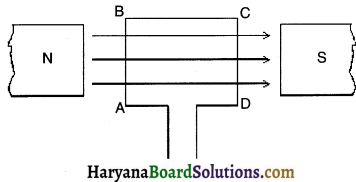(i) An electric current will be induced in the direction from A to B.
(ii) There will be no current induced if the coil is moved in the same direction of magnetic field.

Question 33.
Which are the three component wires of the main power supply that transmit electric current in our homes?
The three component wires are

• live wire (with red insulation)
• neutral wire (with black insulation) and
• Earth wire (with green insulation).

Question 34.
What is the safety measure adopted in the connection of main power supply that is used for current transmission In domestic electric circuits?
A green coloured earth wire is used in the main power supply and is connected to the domestic electric circuits. It is then connected with a metal plate deep in the earth near the house.

Question 35.
How does an earth wire save a user from getting an electric shock?
If a user is working on an appliance where a leakage of current takes place, the current gets transmitted under the Earth’s surface through the earth wire and thus saves the user from getting an electric shock.Question 36.
Give two reasons for using a parallel connection In domestic electric circuits.
(i) Same voltage of the main power supply is available for each appliance connected with the supply.
(ii) Even if one of the appliance stops working there will be no effect in operating other appliances.

Question 37.
Mention any two circumstances under which an extremely high amount of electric current may flow through the main power supply of domestic wiring.
Transmission of a very high current may take place under the occurrence of a ‘short circuit’ or overloading.

Question 38.
What precautions should be taken to avoid overloading of domestic electric circuits?
(i) High power rating devices should be connected with 15A power line.
(ii) Multiple high power rating devices should not be switched on at the same time.
(iii) Multiple high power rating devices should not be connected in a single socket.

Question 39.
Name two safety device commonly used in domestic electric circuits and appliances.
(i) Electric fuse and
(ii) Earth wire

Question 40.
What should be considered while connecting a fuse wire with an appliance so that it can protect the appliance from fluctuating current?
Care should be taken that the fuse wire should have the capacity of drawing current with the same or slightly higher magnitude than that of the appliance.Question 41.
A fuse wire of 5A is connected with an appliance of 200W power rating. If the voltage source is 240V, will the fuse wire be able to protect the connected appliance from fluctuating voltage?
P = 200W, V = 240V
We know that P = VI
∴ $$I=\frac{P}{V}=\frac{200}{240} \quad I=0.83 \mathrm{~A}$$
Hence the fuse wire of 5A will not be able to protect this appliance because the fuse wire will not burn until the current > 5 A passes through circuit which is very high for this appliance.

Fill in the blanks:

1. Like poles of a magnet ……….. each other whereas unlike poles ………….. each other.

2. Magnetic field is a physical quantity which requires ………… and ………….. to define it.

3. If a wire is bent circularly and electric current is passed through it, the magnetic effect of current ………

4. Magnetic field strength of a coil is …………. proportional to the number of turns and ……….. proportional to the distance from the coil.

5. If you are holding a current carrying conductor in right hand such that the current is in the direction of your thumb pointing in upward direction. Then, the magnetic field produced will be in ……… direction if viewed from the top.6. Magnetic field lines of a current carrying …………. resembles to the field lines of a bar magnet.

7. If a solenoid has 14 turns, the magnetic field resulting by it is times stronger than the magnetic field resulting by each circular coil.

8. ………….rule helps in identifying the direction of force that a current carrying conductor experiences when kept in a magnetic field.

9. In Fleming’s left hand rule, the forefinger should point the direction of …………….

10. Electric motor is an appliance which converts ………….. energy into ……………… energy.

11. In an electric motor the direction of current changes at every ………….

12. In a motor, a loop containing many turns is arranged on axis. Such kind of arrangement is called ……………..

13. A magnetic field induces electric current in a stationary conductor kept in it.

14. When the motion of conductor is in a direction to the magnetic field, a large amount of induced electric current is obtained.15. In Fleming’s right hand rule, the fore finger shows the direction of …………….

16. A generator having …………….. produces direct current.

17. A DC generator works on the principle of …………….

18. The difference between …………. and ……………. is that the former remains unidirectional with time whereas the later changes its direction with time.

19. In India, the electric current transmitted for domestic usage, changes direction after every …………… second.

20. Frequency of direct current is ………………… Hz.

21. In India, the potential difference between the live wire ar\\$ is kept at volt in main power supply of domestic wiring.

22. In our homes, the devices such as air conditioners are connected with a ampere …………….. (number) connection whereas devices such as televisions are connected with ampere (number) connection.

23. A fuse is always connected in …………… connection with a ………….. wire in the main power supply.24. …………… wire is generally …………… in colour and is connected with the metallic body of an appliance.

True OR False:

1. The magnetic field lines can be seen approaching towards each other and intersecting at the two poles of a bar magnet. — False
2. The magnetic field strength of a current carrying conductor is directly proportional to the electric current passing through it. — True
3. The magnetic field inside a long circular coil carrying current will be parallel straight lines. — True
4. A wire Is placed perpendicularly on top of a horizontal plane, if a magnetic field is formed in anti-clockwise direction around the wire, it means
that negative terminal of the battery is connected to the top end of conductor. — True
5. A current carrying solenoid has a magnetic field resembling that of a horse-shoe magnet due to its shape. — False
6. It is observed that the force experienced by a conductor is maximum, when a magnetic field is In the same direction as that of current flowing in it as both the fields will add up to resultant force. — False
7. The device which works on the principle of electromagnetic Induction is used to convert electrical energy to mechanical energy. — False
8. Split rings in the electric motor act as a device which reverses the flow of electric current flowing through the rectangular coil. — True
9. As per Fleming’s left hand rule, if the forefinger indicates the direction of magnetic field and thumb shows the direction of motion of conductor,
then middle finger will show the direction of induced current. — False
10. In an AC generator, we notice that In one full revolution of the coil, current changes its direction two times. — True
11. An AC generator can be converted into a DC generator it we replace a split ring wifi, a slip ring. — False
12. The current which changes its direction with time is easy to transmit over a long distance without major loss of energy. — True
13. Potential difference between earth wire and neutral wire in our country is kept at 220 V. — FalseMatch the Following:

Question 1.

 A B 1. Right hand thumb rule a. To detect the direction of induced current 2. Fleming’s right hand rule b. To detect the poles of an electromagnet 3. Fleming’s left hand rule c. To detect the direction of magnetic field lines d. To detect the force acting on a conductor

Question 2.

 A B 1. Magnet exerts an equal and opposite force on a current carrying conductor a. Michael Faraday 2. Magnetic field is generated near a current carrying conductor b. Andre Marie Ampere 3. A moving magnet can induce electric current c. James Maxwell d. Hans Christian Oersted